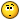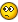Recommended Posts

I'd like to make an easy number cipher, pratically just add N number to the final result

My attempt:

\$sReturn = ""
\$sText = "0123456789"
\$nPos = 3
\$aSplit = StringSplit(\$sText, "")
For \$i = 0 To UBound(\$aSplit) - 1
Select
Case Number(\$aSplit[\$i]) < 7
\$sReturn &= \$aSplit[\$i] + \$nPos
Case Number(\$aSplit[\$i]) = 7
\$sReturn &= 0
Case Number(\$aSplit[\$i]) = 8
\$sReturn &= 1
Case Number(\$aSplit[\$i]) = 9
\$sReturn &= 2
EndSelect
Next
ConsoleWrite(\$sReturn & @CRLF)

I don't know how to make the number start from zero if is bigger the 9, i can't make the case like the example because work only if \$nPos = 3
Thanks

Share on other sites

Please explain a bit more "I don't know how to make the number start from zero if is bigger the 9, i can't make the case like the example because work only if \$nPos = 3"

and exact goal of script.

Monkey's are, like, natures humans.

Share on other sites

Ok

I want to add a number to another number, in the example the position are three, but is a variable and this value can change so:

0 = 3 (0+3)

1 = 4 (1+3)

2 = 5 (2+3)

But when it come the 7

7 = 10 (7+3)

Is wrong, the number are only ten and the max value is 9, so need to start from zero:

7 = 0

8 = 1

9 = 2

So explected string is, if the position to move are three:

0123456789 = 3456789012

Is clear?

Oh, i need to reverse the process tooEdited by MyEarth

Share on other sites

using Mod() could do

\$sReturn = ""
\$sText = "0123456789"
\$nPos = 3
\$aSplit = StringSplit(\$sText, "", 2)
For \$i = 0 To UBound(\$aSplit) - 1
\$sReturn &= Mod(\$aSplit[\$i] + \$nPos, 10)
Next
ConsoleWrite(\$sReturn & @CRLF)

small minds discuss people average minds discuss events great minds discuss ideas.... and use AutoIt....

Share on other sites

ThanksShare on other sites

Wait, and for reverse the processCome back from 3456789012 To 0123456789

Edited by MyEarth

Share on other sites

Wait, and for reverse the processCome back from 3456789012 To 0123456789this could do ...

\$sReturn = ""
\$sText = "0123456789"
\$nPos = 3
\$max = 10 ; (0-9)
;
; forth
\$aSplit = StringSplit(\$sText, "", 2)
For \$i = 0 To UBound(\$aSplit) - 1
\$sReturn &= Mod(\$aSplit[\$i] + \$nPos, \$max)
Next
ConsoleWrite(\$sReturn & @CRLF)
;
; and back
\$aSplit = StringSplit(\$sReturn, "", 2)
\$sReturn = ""
For \$i = 0 To UBound(\$aSplit) - 1
\$sReturn &= Mod(\$aSplit[\$i] - \$nPos + (\$max * (\$aSplit[\$i] < \$nPos)), \$max)
Next
ConsoleWrite(\$sReturn & @CRLF)Edited by Chimp

small minds discuss people average minds discuss events great minds discuss ideas.... and use AutoIt....

Create an account or sign in to comment

You need to be a member in order to leave a comment

Create an account

Sign up for a new account in our community. It's easy!

Register a new account